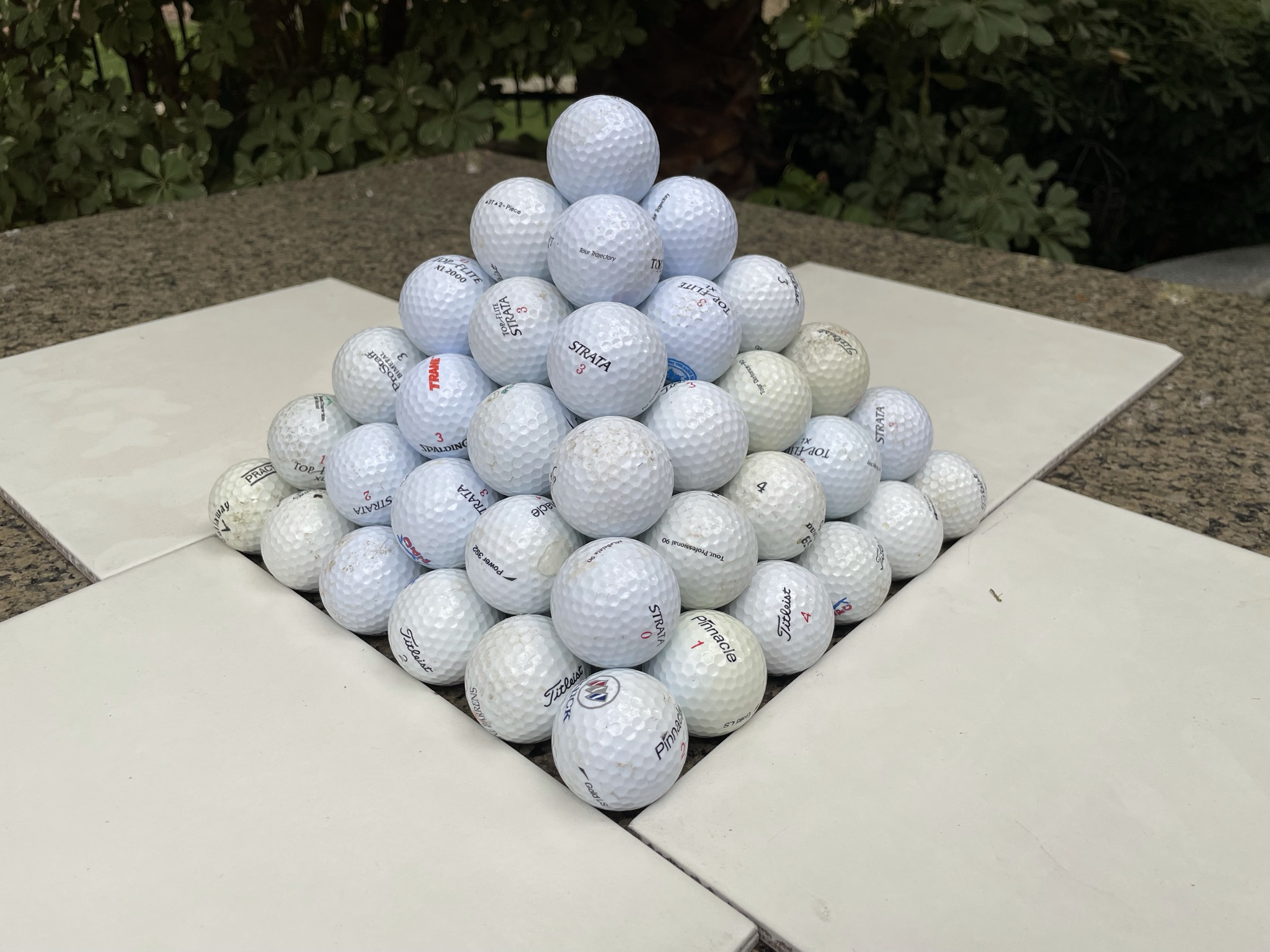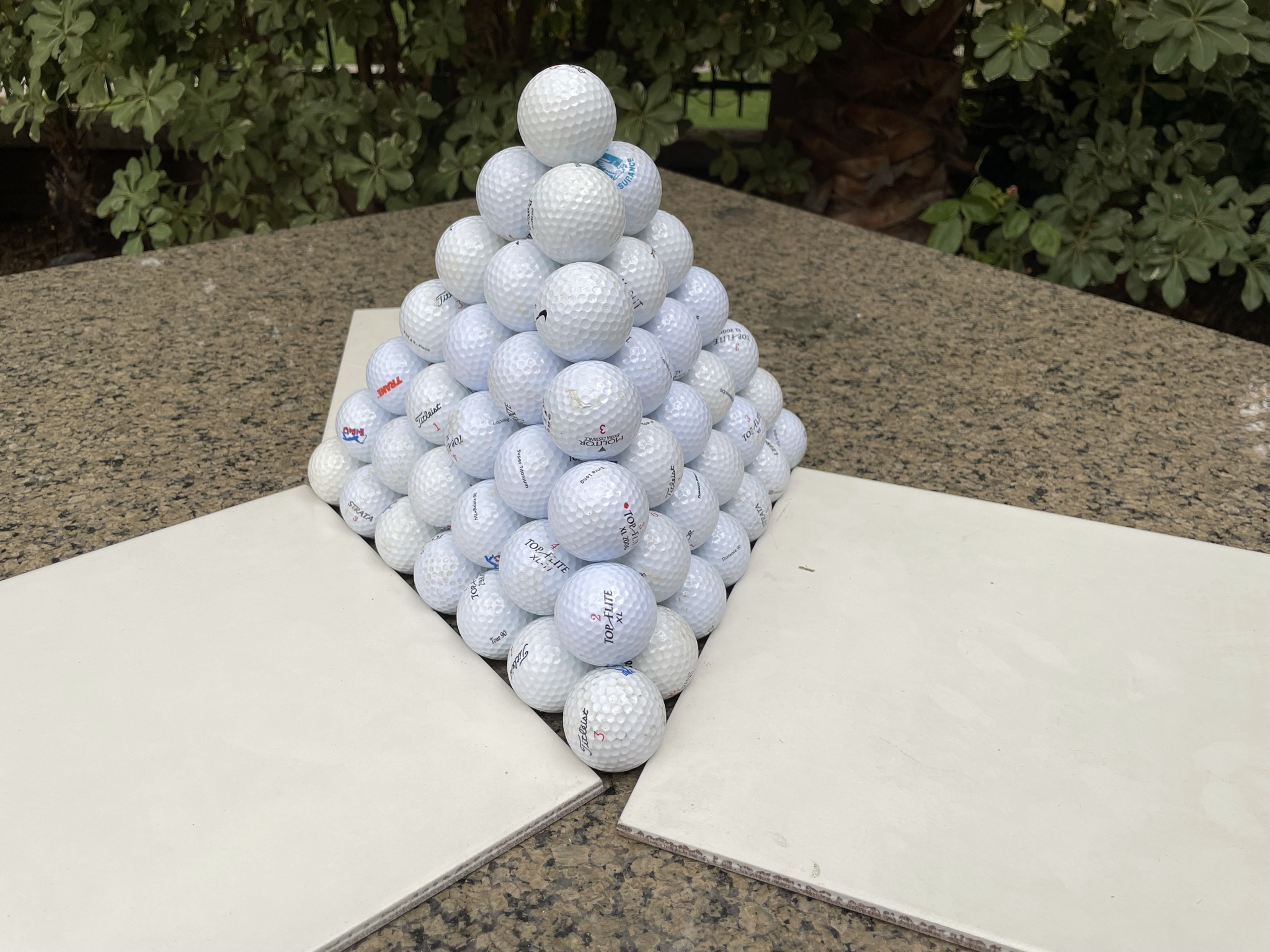## Poll

 I love math!17 votes (50%) Math is great.13 votes (38.23%) My religion is mathology.5 votes (14.7%) Women didn't speak to me until I was 30.2 votes (5.88%) Total eclipse reminder -- 04/08/202411 votes (32.35%) I steal cutlery from restaurants.3 votes (8.82%) I should just say what's on my mind.6 votes (17.64%) Who makes up these awful names for pandas?5 votes (14.7%) I like to touch my face.10 votes (29.41%) Pork chops and apple sauce.8 votes (23.52%)

34 members have voted

WizardJoined: Oct 14, 2009
• Posts: 23444
August 24th, 2021 at 5:49:31 PM permalink
Quote: ssho88

1) x= width of rectangle, y = height of rectangle, 4/x=y/5, xy = 20

2) Entire figure area, A = (4+y) * (5 +x)/2 = (20 + 4x + 5y + xy)/2, and xy = 20

So, A = 20 + 2x + 50/x

dA/dx = 2 - 50/x^2 = 0

x= 5

Minimum A = 20 + 2(5) + 50/5 = 40

What if x=0, y= 0 ? still applicable to your question ?

I agree to both!
It's not whether you win or lose; it's whether or not you had a good bet.
ssho88Joined: Oct 16, 2011
• Posts: 552
August 24th, 2021 at 7:16:37 PM permalink
Quote: Wizard

I agree to both!

What if x=0, y= 0 ? still applicable to your question ?
ThatDonGuyJoined: Jun 22, 2011
• Posts: 4962
August 24th, 2021 at 7:28:07 PM permalink
Quote: ssho88

What if x=0, y= 0 ? still applicable to your question ?

If you want to be pedantic, if x and y = 0, it's no longer a rectangle, but a point. Even if just one of them = 0, it's a line segment.
Ace2Joined: Oct 2, 2017
• Posts: 1070
August 24th, 2021 at 8:51:06 PM permalink
Quote: ThatDonGuy

Who wants a rather lengthy explanation of how to calculate the exact (rational number) solution to these integrals?

I will use the "direct method fire bet" integral in the example:
(
(1 - e^(-165x/3960))^2 * (1 - e^(-264x/3960))^2 * (1 - e^(-375x/3960)) * e^(-375x/3960) * 375/3960
+ (1 - e^(-165x/3960))^2 * ( 1 - e^(-375x/3960))^2 * ( 1 -e^(-264x/3960)) * e^(-264x/3960) * 264/3960
+ (1 - e^(-375x/3960))^2 * (1 - e^(-264x/3960))^2 * (1 - e^(-165x/3960)) * e^(-165x/3960) * 165/3960
) * e^(-2352x/3960) * 2 dx

Let y = e^(-x/3960): the integral becomes
2 *
(
(1 - y^165)^2 * (1 - y^264)^2 * (1 - y^375) * y^375 * 375/3960
+ (1 - y^165)^2 * (1 - y^375)^2 * (1 - y^264) * y^264 * 264/3960
+ (1 - y^375)^2 * (1 - y^264)^2 * (1 - y^165) * y^165 * 165/3960
)
* y^2352 dx

Expand all of the squares, and take 1/3960 out of the sum:
2 / 3960 *
(
(1 - 2 y^165 + y^330) * (1 - 2 y^264 + y^528) * (1 - y^375) * y^375 * 375
+ (1 - 2 y^165 + y^330) * (1 - 2 y^375 + y^750) * (1 - y^264) * y^264 * 264
+ (1 - 2 y^375 + y^750) * (1 - 2 y^264 + y^528) * (1 - y^165) * y^165 * 165
)
* y^2352 dx

I will not bore you with calculating the expansion (hint: use Excel, and a lot of cutting and pasting), but this is:
1 / 1980 *
(
165 y^2517
+ 264 y^2616
- 165 y^2682
+ 375 y^2727
- 528 y^2781 - 330 y^2781
- 264 y^2880
- 750 y^2892 - 330 y^2892
+ 264 y^2946 + 330 y^2946
- 750 y^2991 - 528 y^2991
+ 528 y^3045 + 165 y^3045
+ 375 y^3057 + 330 y^3057
- 375 y^3102
+ 1500 y^3156 + 1056 y^3156 + 660 y^3156
- 264 y^3210 - 165 y^3210
+ 375 y^3255 + 528 y^3255
+ 750 y^3267 + 165 y^3267
- 750 y^3321 - 528 y^3321 - 660 y^3321
+ 750 y^3366 + 264 y^3366
- 750 y^3420 - 1056 y^3420 - 330 y^3420
- 375 y^3432 - 165 y^3432
- 1500 y^3531 - 528 y^3531 - 330 y^3531
+ 375 y^3585 + 528 y^3585 + 330 y^3585
- 375 y^3630 - 264 y^3630
+ 750 y^3696 + 264 y^3696 + 330 y^3696
+ 750 y^3795 + 528 y^3795 + 165 y^3795
- 375 y^3960 - 264 y^3960 - 165 y^3960
)

"Now what?"
d/dx (e^(nx)) = e^(nx) / n
y^n = e^(x * (-n/3960)), so d/dx (y^n) = e^(x * (-n/3960)) * (-3960/n)
For positive n, at x = +INF, this is 0; at x = 0, this is -3960/n, so the integral over x = 0 to +INF = 3960/n

Replace each "a y^b" with "3960 * b / a"; you can take 3960 out of each numerator and multiply the 1/1980 term by 3960 to get 2.
The solution becomes
2 *
(
165 / 2517
+ 264 / 2616
- 165 / 2682
+ 375 / 2727
- 858 / 2781
- 264 / 2880
- 1080 / 2892
+ 594 / 2946
- 1278 / 2991
+ 693 / 3045
+ 705 / 3057
- 375 / 3102
+ 3216 / 3156
- 429 / 3210
+ 903 / 3255
+ 915 / 3267
- 1938 / 3321
+ 1014 / 3366
- 2136 / 3420
- 540 / 3432
- 2358 / 3531
+ 1233 / 3585
- 639 / 3630
+ 1344 / 3696
+ 1443 / 3795
- 804 / 3960
)

Easy, right?
The sum is done one term at a time (i.e. start with the first two, then add the third, then add the fourth, and so on), using:
a / b + c / d = (ad + bc) / bd
No, you can't do this in Excel, as you will soon run out of digits of precision.

It�s very useful to see that some of these can be hashed out manually. However, if you need to take the integral of, for instance, 1-(1-(1+(x/6)+(x/6)^2/2+ (x/6)^3/6+ (x/6)^4/24+ (x/6)^5/120)*e^(-x/6))^6 or 1-(1-1/e^(x/38))^38, would it be feasible to do it manually ?

Incidentally, the result is the expected number of rolls to hit all six sides of a single die at least six times and the expected number of spins to hit all 38 numbers on a roulette wheel at least once, respectively
It�s all about making that GTA
WizardJoined: Oct 14, 2009
• Posts: 23444
August 25th, 2021 at 2:59:36 AM permalink
Quote: ssho88

What if x=0, y= 0 ? still applicable to your question ?

No, because it wouldn't be inscribed in the triangle.
It's not whether you win or lose; it's whether or not you had a good bet.
ThatDonGuyJoined: Jun 22, 2011
• Posts: 4962
August 25th, 2021 at 10:17:01 AM permalink
Quote: Ace2

It�s very useful to see that some of these can be hashed out manually. However, if you need to take the integral of, for instance, 1-(1-(1+(x/6)+(x/6)^2/2+ (x/6)^3/6+ (x/6)^4/24+ (x/6)^5/120)*e^(-x/6))^6 or 1-(1-1/e^(x/38))^38, would it be feasible to do it manually ?

Who said anything about "feasible"? Just integrating something like x e^(-x/6) is a pain.
Integrate by parts:
u = x; dv = e^(-x/6) dx
du = dx; v = -6 e^(-x/6)
The integral = -6x e^(-x/6) - the integral of -6 e^(-x/6) dx = -6x e^(-x/6) + 6 (-6 e^(x/6)) = -(6x + 36) e^(-x/6) = -6 (x + 6) / e^(x/6)
As x approaches positive infinity, the numerator and denominator approach positive infinity, so apply L'Hopital's Rule; the limit of 1 / (6 e^(x/6)) = 0, so the definite integral over x from 0 to positive infinity = 0 - (-6 (0 + 6) / e^0)) = 36.
teliotJoined: Oct 19, 2009
• Posts: 2255
August 26th, 2021 at 9:44:30 AM permalink
What is the largest solution you can find of the equation:

1 + 2 + 3 + ... + N = (N+1) + (N+2) + ... (N+K)?

I have solutions so far for N = 2, 14, 84, 492, 2870, 16730 and 97512.
Poetry website: www.totallydisconnected.com
teliotJoined: Oct 19, 2009
• Posts: 2255
August 26th, 2021 at 10:42:38 AM permalink
Quote: teliot

What is the largest solution you can find of the equation:

1 + 2 + 3 + ... + N = (N+1) + (N+2) + ... (N+K)?

I have solutions so far for N = 2, 14, 84, 492, 2870, 16730 and 97512.

And now I just ruined it for myself by looking up the sequence on OEIS.org :) Well, it was fun anyway for a few minutes.
Poetry website: www.totallydisconnected.com
charliepatrickJoined: Jun 17, 2011
• Posts: 2444
August 26th, 2021 at 11:47:18 AM permalink
Quote: Wizard

...I agree to both!

I didn't see the solution beforehand...
Just coming to this but happened to fall over the solution quite quickly. The way I saw it was to use similar triangles which gives 4/x = y/5 leading to xy=20.
The second idea is then to flip the 5-wide triangle to lie on top of the diagonal line. Construct a rectangle 4x5 (to the right of the 4-side). Where x is less than 5, the top triangle extends below this rectangle. Hence the total area of the original triangle exceeds 40. Similar logic applies where x exceeds 5 except the 4-side triangle extends beyond the rectangle. Hence 40 is the minimum.
WizardJoined: Oct 14, 2009
• Posts: 23444
August 31st, 2021 at 7:39:40 PM permalink
Which method of stacking cannonballs is the most efficient -- a pyramid with a square base or triangular?For full credit, I'd like to the degree of efficiency (meaning ratio of the volume of cannonballs to to the volume of the whole pyramid), both ways, as the number of cannonballs approaches infinity?

There will probably be an extra credit problem coming up, but one thing at a time.
It's not whether you win or lose; it's whether or not you had a good bet.